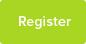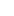# Tutor Hunt Questions

Express 3sinθ+5sinθ in the form Rsin(θ+α)
3 years ago

Hi there, Did you mean either 3sinθ + 5cosθ? Or 3cosθ + 5sinθ? Chris
29/02/2016 14:03:07 | comment by Christopher
Are you sure that the question gives 3sinθ+5sinθ, and not 3sinθ+5cosθ?
29/02/2016 20:32:34 | comment by [Deleted Member]
I changed the question to what I think was meant.
29/02/2016 22:29:14 | comment by David
3 of something plus 5 of something is just 8 of something So 3sin⁡θ + 5sin⁡θ = 8sin⁡θ
09/08/2016 14:26:05 | comment by Michael

Please enter your response to the question below. The student will get a notification as soon your response has been approved by our moderation team.

As I believe your original question was
"Express 3sinθ + 5cosθ in the form Rsin(θ+α)"

this is how you would work it out:

1. Expand Rsin(θ+α) differently using the double angle formula:

Rsinθcosα + Rsinαcosθ

2. (Rcosα)= 3 and (Rsinα)= 5.

Answered by Imogen | 2 years ago
3 of something plus 5 of something is just 8 of something
So 3sin⁡θ + 5sin⁡θ = 8sin⁡θ
Answered by Michael | 2 years ago
3SinW + 5SinW = 8Sin(W + 0)
Answered by Raji | 3 years ago
(5+3) sin(theta+alpha)
If we decide to call Sinθ, X instead, just to make things look simpler, we can write the equation as this. Sinθ=X so 3X + 5x.

Now we know 3X just equals X+X+X and 5x just equals X+X+X+X+X, so if we add them together we get 8X!

If we think about what we did in the beginning, we can just change X back into Sinθ, like this, 8Sinθ.

The questions asks for the form Rsin(θ+a), so all we have to do is look at our answer, which is 8sinθ, and see what R and a equal. R is clearly 8, and theta has nothing added to it, so a must be zero.

Hope this helps, if you have any questions feel free to ask!
Hi Matt. I think you made an error when posting it, one factor should be in cos(theta).
I think `α` is meant for the periodicity, so its 180 degree. So the answer is
3*sin⁡θ + 5*sin⁡θ = 8*sin(θ+180)

BW
T
3sinW +5sinW = Rsin(W + a): I take it that the question is :

3sinW + 5cosW = Rsin(W + a)

So Rsin(W + a) = R(sinW*cosa + cosW*sina)

= (Rcosa)sinW + (Rsina)cosW

Compare 3sinW + 5cosW with (Rcosa)sinW + (Rsina)cosW

Clearly Rsina = 5

Rcosa = 3
________________________________________________________________
so (Rsina)*(Rsina) + (Rcosa)*(Rcosa) = 3*3 + 5*5

then R*R( sina*sina + cosa *cosa) = 34 NB. ( sina*sina + cosa *cosa) = 1

thus R*R = 34

R = square root of 34 =1.73

______________________________________________________________-

Rsina/Rcosa =5/3

sina/cosa = 5/3

tana = 5/3

a = inverse tan (5/3) = 59.0 degrees

________________________________________________________________
so 3sinW + 5CosW = 1.73sin( W + 59.0)
3sinΘ + 5sinΘ express to Rsin(θ+α)

Rsin(θ + α) can be expand to Rsinθ + Rsinα

let x = sinθ
so 3x + 5x = 8x
replace x for sinθ
3sinθ + 5sinθ = 8sinθ

In the form Rsin(θ + α) = Rsinθ + Rsinα where R is 8 so what is sinα?
if sinα is equals zero what is α?

Rsinθ + Rsinα = 8sinθ + 8sinα

if sinα = 0
α = 〖sin〗^(-1)0 = 0 or 180 because sin(0) = 0 or sin(180) = 0

sin(α) = sin(0) or sin(180) where α is neither 0 or 180

Rsinθ + Rsinα = Rsin(θ + α) where R = 8

so

8sinθ + 8sinα = 8sin(θ + 0) or 8sin(θ + 180)

Hope this helps?

I think you have made a mistake with your question, I think you meant to write 3sinθ+5cosθ. If that`s what you meant then if you expand Rsin(θ+α)=Rsinθcosα +Rcosθsinα
Then equate coefficients:
3sinθ=Rsinθcosα therefore 3=Rcosα
and 5cosθ = Rcosθsinα therefore 5= Rsinα
dive the last two equations Rsinα / Rcosα =tanα = 5/3
square and add you get 9+25 = R squared (since square of sine + square of cos is 1), so R=root of 34

If your original question was correct then 3sinθ+5sinθ = 8sinθ
so R=8 and α = 0